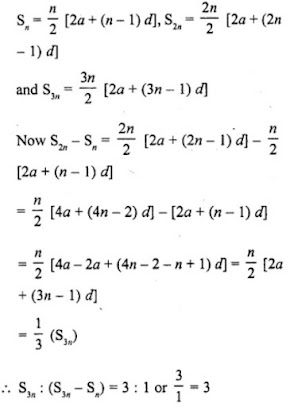# RD Sharma Solutions for Class 10 Maths Chapter 9 Arithmetic Progression MCQs and VSAQs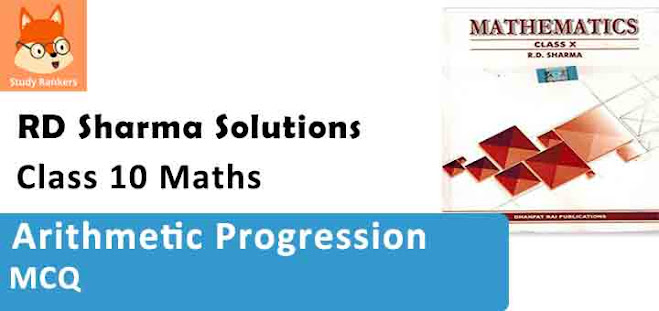#### VSAQs

1. Define an arithmetic progression.

Solution

A sequence a1, a2, a3, …, an is called an arithmetic progression of then exists a constant d
Such that a2 – a1 = d, a3 – a2 = d, …… an – an-1 = d
and so on and d is called common difference

2. Write the common difference of an A.P. whose nth term is an = 3n + 7.

Solution

an = 3n + 7
a1 = 3×1 + 7 = 3 + 7 = 10
a2 = 3×2 + 7 = 6 + 7 = 13
a3 = 3×3 + 7 = 9 + 7 = 16
d = a3 – a2 or a2 – a1 = 16 – 13 = 3 or 13 – 10 = 3

3. Which term of the sequence 114, 109, 104, … is the first negative term ?

Solution

Sequence is 114, 109, 104, …..
Let an term be negative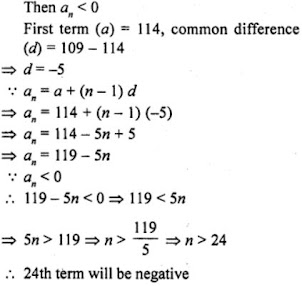4. Write the value of a30 – a10 for the A.P. 4, 9, 14, 19, ……

Solution

In the A.P. 4, 9, 14, 19,....
First term (a) = 4
Common difference (d) = 9 - 4 = 5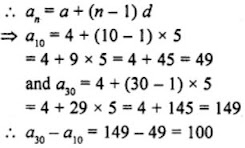5. Write 5th term from the end of the A.P. 3, 5, 7, 9,…, 201.

Solution

A.P. is 3, 5, 7, 9, ..., 201
Here, first term (a) = 3
Common difference (d) = 5 - 3 = 2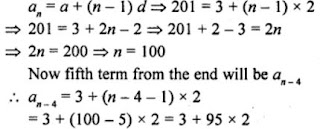= 3 + 190 = 193
5th term from the end = 193

6. Write the value of x for which 2x, x + 10 and 3x + 2 are in A.P.

Solution

2x, x+10 and 3x+2 are in A.P.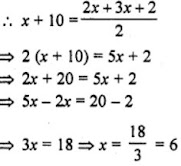7. Write the nth term of an A.P. the sum of whose n terms is Sn.

Solution

Sum of n terms = Sn
Let a be the first term and d be the common difference an =Sn – Sn-1

8. Write the sum of first n odd natural numbers.

Solution

The first n odd natural numbers are 1, 3, 5, 7,....
Here, a = 1, d = 3 - 1 = 2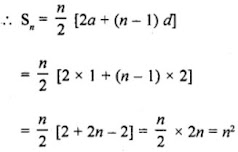9. Write the sum of first n even natural numbers.

Solution

First n even natural numbers are
2, 4, 6, 8, ……
Here a = 2, d = 2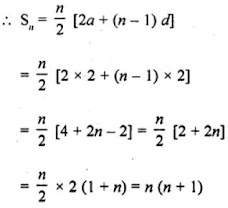10. If the sum of n terms of an A.P. is Sn = 3n² + 5n. Write its common difference.

Solution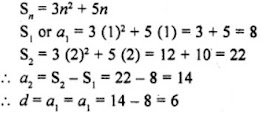11. Write the expression for the common difference of an A.P. Whose first term is a and nth term is b.

Solution

First term of an A.P. = a
and an = a + (n – 1) d = b
Subtracting, b – a = (n – 1) d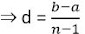12. The first term of an A.P. is p and its common difference is q. Find its 10th term.

Solution

First term of an A.P. (a) = p
and common difference (d) = q
a10 = a + (n – 1) d
= p + (10 – 1) q = p + 9q

13. For what value of p are 2p + 1, 13, 5p – 3 are three consecutive terms of an A.P.?

Solution

∵ 2p + 1, 13, 5p - 3 are consecutive terms of an A.P.
∴ c.d. = 13 - 2p - 1
⇒ 5p + 2p = 13 - 1 + 13 + 3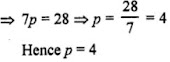14. If 4/5, a, 2 are three consecutive terms of an A.P., then find the value of a.

Solution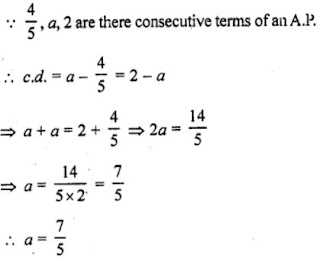15. If the sum of first p term of an A.P. is ap² + bp, find its common difference.

Solution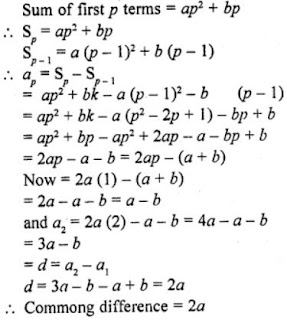16. Find the 9th term from the end of the A.P. 5, 9, 13, …, 185.

Solution

Here, first term, a = 5
Common difference, d = 9 – 5 = 4
Last term, l = 185
nth term from the end = l – (n – 1) d
9th term from the end = 185 – (9 – 1) 4 = 185 – 8×4 = 185 – 32 = 153

17. For what value of k will the consecutive terms 2k + 1, 3k + 3 and 5k – 1 form on A.P.?

Solution

(3k + 3) – (2k + 1) = (5k – 1) – (3k + 3)
⇒ 3k + 3 – 2k – 1 = 5k – 1 – 3k – 3
⇒ k + 2 = 2k – 4
⇒ 2k – k = 2 + 4
⇒ k = 6

18. Write the nth term of the A.P.
1/m, 1+m/m, 1+2m/m,....

Solution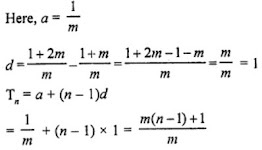### MCQs

1. If 7th and 13th terms of an A.P. be 34 and 64 respectively, then its 18th term is

(a) 87
(b) 88
(c) 89
(d) 90

Solution

(c)
7th term (a7) = a + 6d = 34
13th term (a13) = a + 12d = 64
Subtracting, 6d = 30 => d = 5
and a + 12×5 = 64
⇒ a + 60 = 64
⇒ a = 64 – 60 = 4
18th term (a18) = a + 17d = 4 + 17×5 = 4 + 85 = 89

2. If the sum of p terms of an A.P. is q and the sum of q terms is p, then the sum of (p + q) terms will be

(a) 0
(b) p – q
(c) p + q
(d) – (p + q)

Solution

(d)
Sum of p terms = q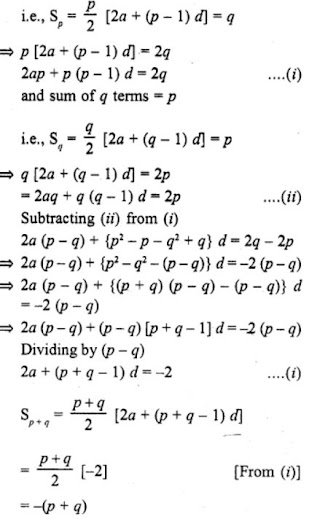3. If the sum of n terms of an A.P. be 3n2 + n and its common difference is 6, then its first term is

(a) 2
(b) 3
(c) 1
(d) 4

Solution

(d)
Sum of n terms of an A.P. = 3n2 + n
and common difference (d) = 6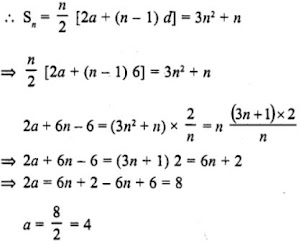4. The first and last terms of an A.P. are 1 and 11. If the sum of its terms is 36, then the number of terms will be

(a) 5
(b) 6
(c) 7
(d) 8

Solution

(b)
First term of an A.P. (a) = 1
Last term (l) = 11
and sum of its terms = 36
Let n be the number of terms and d be the common difference, then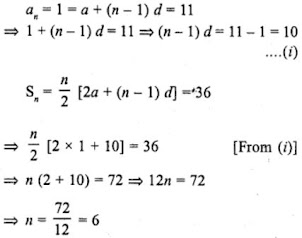5. If the sum of n terms of an A.P. is 3n2 + 5n then which of its terms is 164 ?

(a) 26th
(b) 27th
(c) 28th
(d) none of these

Solution

(b)
Sum of n terms of an A.P. = 3n2 + 5n
Let a be the first term and d be the common difference.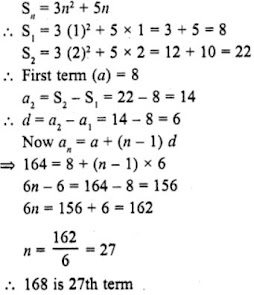6. If the sum of it terms of an A.P. is 2n2 + 5n, then its nth term is

(a) 4n – 3
(b) 3n – 4
(c) 4n + 3
(d) 3n + 4

Solution

(c)
Let a be the first term and d be the common difference of an A.P. and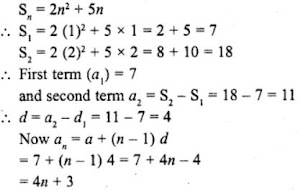7. If the sum of three consecutive terms of an increasing A.P. is 51 and the product of the first and third of these terms is 273, then the third term is :

(a) 13
(b) 9
(c) 21
(d) 17

Solution

(c)

Let three consecutive terms of an increasing A.P. be a-d, a, a+d where a is the first term and d be the common difference.
a-d + a + a+d = 51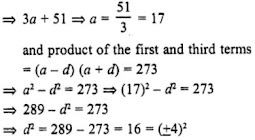∴ d = ±4
∵ The A.P. is increasing.
∴ d = 4
Now, third term = a + d
= 17 + 4 = 21

8. If four numbers in A.P. are such that their sum is 50 and the greatest number is 4 times the least, then the numbers are

(a) 5, 10, 15, 20
(b) 4, 10, 16, 22
(c) 3, 7, 11, 15
(d) None of these

Solution

(a)
4 numbers are in A.P.
Let the numbers be
a – 3d, a – d, a + d, a + 3d
Where a is the first term and 2d is the common difference
Now their sum = 50
a – 3d + a – d + a + d + a + 3d = 50
and the greatest number is 4 times the least number
a + 3d = 4 (a – 3d)
⇒ a + 3d = 4a – 12d
⇒ 4a – a = 3d + 12d
⇒ 3a = 15d
⇒ a = 5d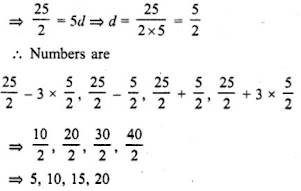9. Let S denotes the sum of n terms of an A.P. whose first term is a. If the common difference d is given by d = Sn – k Sn-1 + Sn-2 then k =

(a) 1
(b) 2
(c) 3
(d) None of these

Solution

(b)

Sn is the sum of n terms of an A.P.
a is its first term and d is common difference.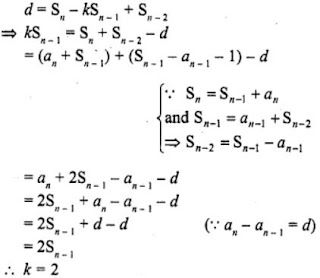10. The first and last term of an A.P. are a and l respectively. If S is the sum of all the terms of the A.P. and the common difference is given by:
l2 - a2/k-(l+a) then k =

(a) S
(b) 2S
(c) 3S
(d) None of these

Solution

(b)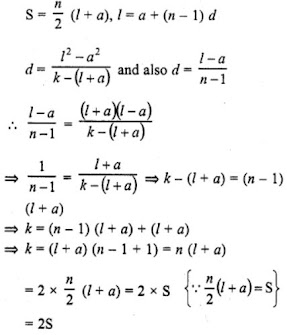11. If the sum of first n even natural number is equal to k times the sum of first n odd natural numbers, then k =

(a) 1/n
(b) n-1/n
(c) n+1/2n
(d) n+1/n

Solution

(d)

Sum of n even natural number = n(n+1)
and sum of n odd natural numbers = n2
∴ n(n+1) = kn2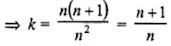12. If the first, second and last term of an A.P. are a, b and 2a respectively, its sum is:

(a) ab/2(b-a)
(b) ab/b-a
(c) 3ab/2(b-a)
(d) None of these

Solution

(c)
First term (a1) = a
Second term (a2) = b
and last term (l) = 2a
∴ d = second term = first term = b-a
∵ l = an + (n-1)d
⇒ 2a = a + (n-1) (b-a)
⇒ (n-1)(b-a) = a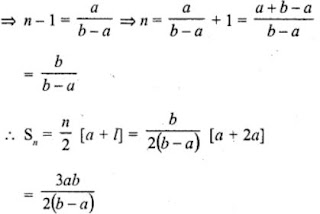13. If S1 is the sum of an arithmetic progression of ‘n’ odd number of terms and S2 is the sum of the terms of the series in odd places, then S1/S2 =

(a) 2n/n+1
(b) n/n+1
(c) n+1/2n
(d) n-1/n

Solution

(a)
Odd numbers are 1, 3, 5, 7, 9, 11, 13,.... n
S1 = Sum of odd numbers = n2
S2 = Sum of numbers at odd places 3, 7, 11, 15, ....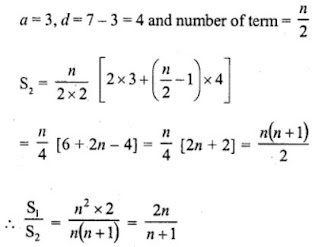14. If in an A.P., Sn = n2p and Sm = m2p, where S denotes the sum of r terms of the A.P., then Sp is equal to

(a) 1/2 p3
(b) mnp
(c) p3
(d) (m + n) p2

Solution

(c)

Sn = n2p, Sm = m2p
Sr = r2p and Sp = p2q = p3
Hence, Sp = p3

15. If Sn denote the sum of the first n terms of an A.P. If S2n = 3Sn, then S3n : Sn is equal to

(a) 4
(b) 6
(c) 8
(d) 10

Solution
(b)

Sn = Sum of n terms of an A.P. and S2n = 3Sn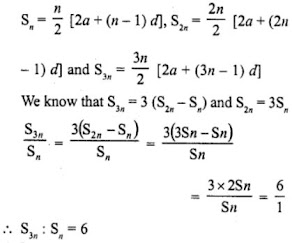16. In an AP, Sp = q, Sq = p and S denotes the sum of first r terms. Then, Sp+q is equal to

(a) 0
(b) – (p + q)
(c) p + q
(d) pq

Solution

(c) In an A.P. Sp = q, Sq = p
Sp+q = Sum of (p + q) terms = Sum of p term + Sum of q terms = q + p

17. If Sn denotes the sum of the first r terms of an A.P. Then, S3n : (S2n – Sn) is

(a) n
(b) 3n
(b) 3
(d) None of these

Solution

(c)